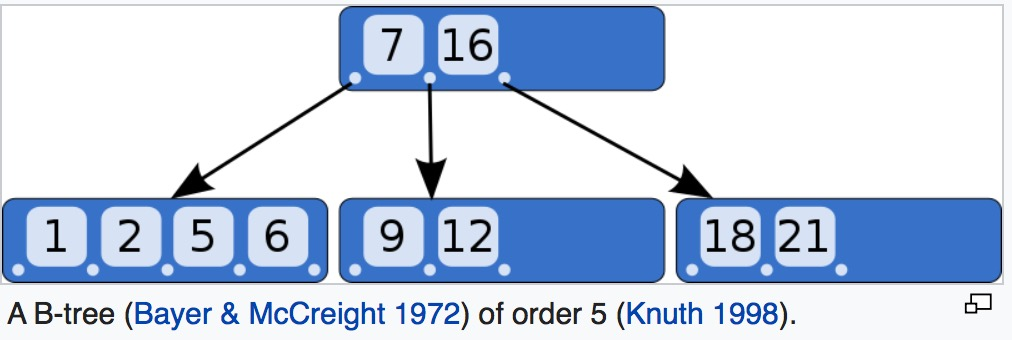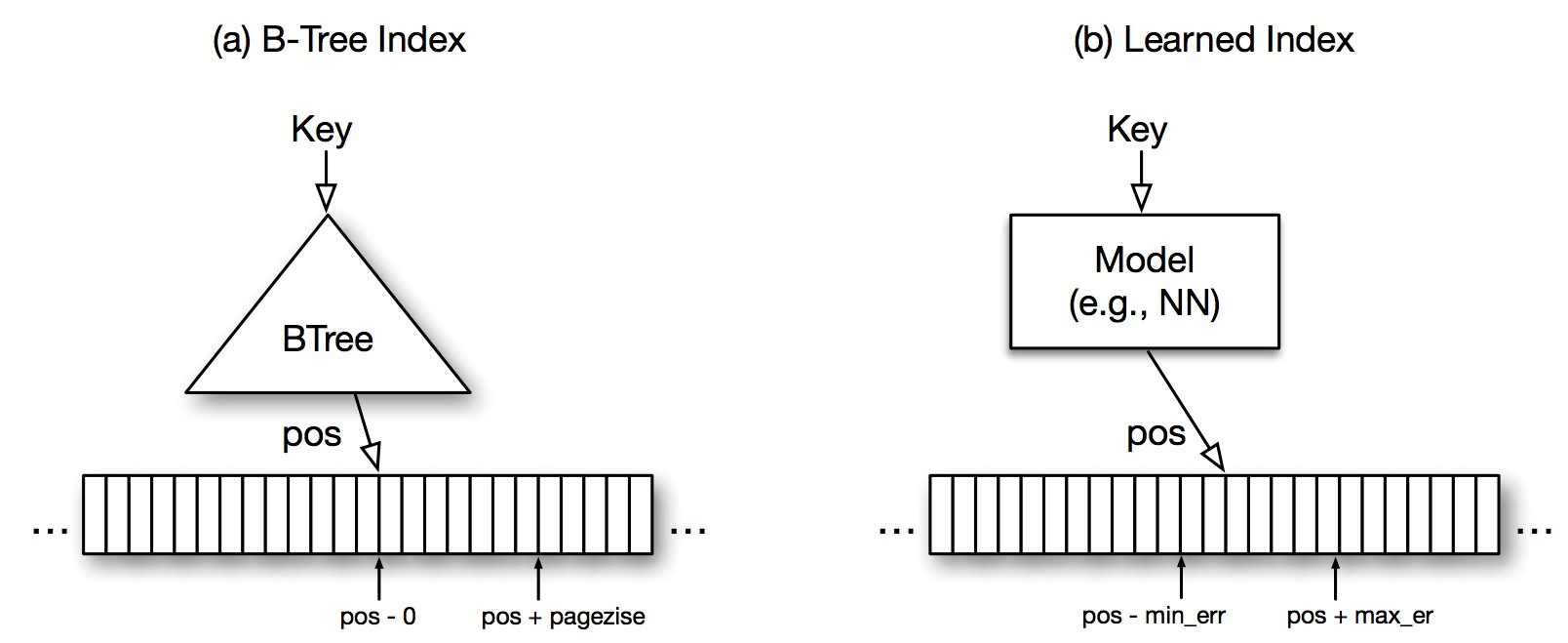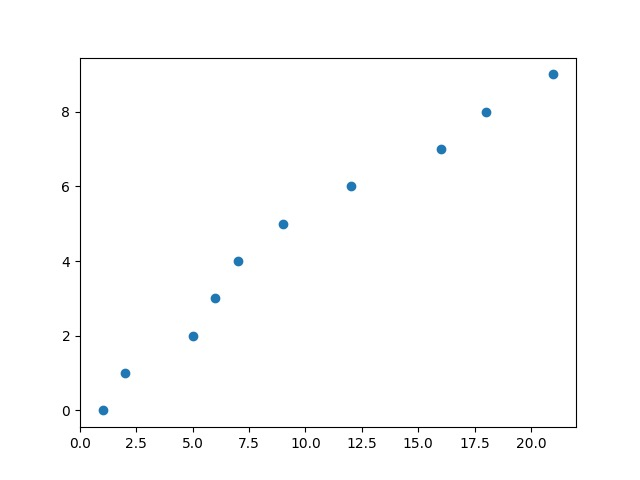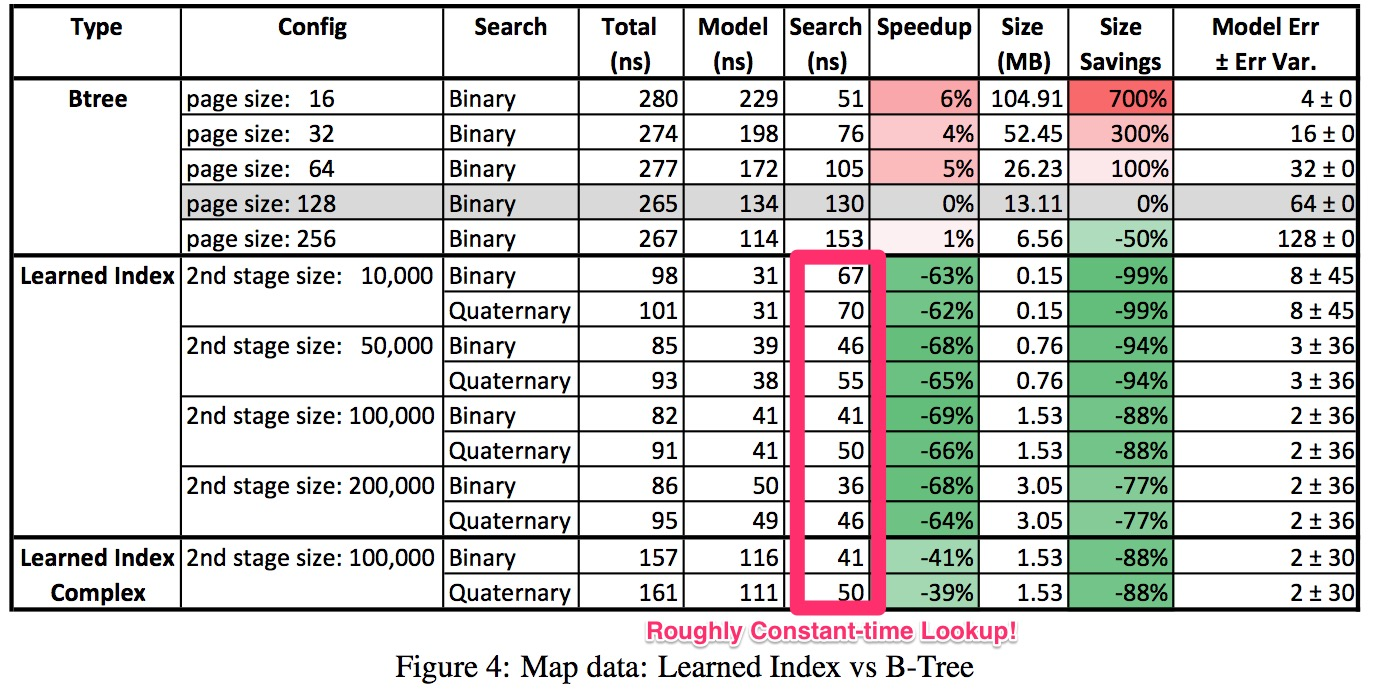# Paper Review: The Case for Learned Index Structures

Alex Egg,

Kraska, Tim, et al. “The Case for Learned Index Structures.” arXiv preprint arXiv:1712.01208 (2017).

## TLDR

Normal DB indexes are designed to be general-purpose so that they give predictable performance no matter the data you insert. The paper basically describes a more efficient way to look up data by using an index that’s tailored to the specific dataset it’s built over. It’s expected that you’d be able to get a performance increase by doing this. In more detail, they use neural nets to learn an approximation of the distribution of the data and use that to look up the rough position of each key faster than usual. They still use traditional structures for the “last-mile” which is not so easily learned. You could accomplish the same thing without NNs by using anything that can approximate the distribution of the data. E.g. a histogram would work for some cases, and you could do some PCA and normalization first to deal with more cases. NNs have the advantage that they can learn more complex distributions.

Another paper one of the powerhouse research groups in Google. This paper shows an applied ML application which aims to replace the good-ol’ workhorses of relational databases: indexes, namely the b-tree. They make claims that their solution which uses a regression has faster lookup times and better storage characteristics.

But first let’s review what an index is in a Relational Database:

## Traditional Indexes (B-trees)

A B-tree is a standard tree data structure which self-balances (thus B-tree) and keeps data sorted to allow most operations (read, insert, delete) to happen in log time.

Imagine we have this list of age data:

data = [16, 9, 5, 2, 21, 1, 6, 18, 7, 12]


If we wanted to find all items over 18 yo we’d have to search the whole list (10 operations in total). Maybe if we sort it we can use a more sophisticated search:

data = [1, 2, 5, 6, 7, 9, 12, 16, 18, 21]


Now we can split the list in two equal parts and then check if our query is in the left or right side and repeat that operation until we find it. This is essentially what we do w/ the B-tree:Figure 1: B-tree example from wikipedia

Now if we want to find where 18 is in the we can find it in only 1 operation. It will return that it is in the 8th position of the array.

### Indexes

Indexes are a vital component database systems, otherwise most search operations would be exhaustive. Indexes, particularly, a T-tree based index allows you to cut down search time from $O(n)$ to $O(logn)$. Indexes also have overhead: As far as storage goes, you have to keep a reference to every item in the database an as far as updating goes there is a computational overhead in rebuilding the index on inserts.

So now when a user queries for a particular item in a table and column, instead of scanning the whole table, the system can walk the tree and find it in a fraction of the time.

A similar approach is used in most non-fiction books: terms and concepts that are frequently looked up by readers are collected in an alphabetic index at the end of the book. The interested reader can scan the index relatively quickly and flip to the appropriate page(s), rather than having to read the entire book to find the material of interest. Just as it is the task of the author to anticipate the items that readers are likely to look up, it is the task of the database programmer to foresee which indexes will be useful.

## Learned Indexes (Regressions)

When traditional databases build the indexes they treat the data as opaque and apply the indexes blindly w/o making any assumptions about the data. Maybe making some inferences about the data distribution could be beneficial? Indexes are very general purpose to fit the widest variety of use-cases. However, what if they had some incites into the data, could they achieve higher performance?

Can you approximate a B-tree using a regression? A B-tree takes a key and returns the position of the record. Can we train a regression of keys against their positions?

The simple example they use in the beginning is of a column w/ numerical data. Say you want to do a query like this:

SELECT * from table
WHERE age > 18


Where the data is the sorted list data above.

If the age column has a B-tree index, it will traverse the tree in Figure 1 to return the position that fits the criteria, in this case position 8 which references 21.

However, if this was a regression, train all the keys (tuples) against the positions. Then to process the query above you would evaluate the linear combination:

where $w$ and $b$ are the learned weights and bias and $x$ is your query key, 18 in this case. The function $y$ would return the position in $a$ where the item $x$ can be found: 8.

data =np.reshape([1,2,5,6,7,9,12,16,18,21], (10,1))
positions=np.reshape([0,1,2,3,4,5,6,7,8,9], (10,1))

from sklearn.linear_model import LinearRegression
model = LinearRegression()
model.fit(data, positions)
model.coef_
model.intercept_
model.predict([])


Output:

array([[ 0.43680076]]) # weight
array([ 0.26303261]) # bias
array([[ 8.12544632]]) # position in array


In the paper they illustrate this ides as:Figure 2: Visual analogy of b-tree as a regression model

It’s an interesting idea, that I have yet to fully explore, but it does come into play regarding the specific distribution of your data. See the visualisation of the regression from above:Figure 3: Scatter of data against position. Data is x axis and position in array is y axis.

This is a simple example where the distribution of positions is easily modelled by a linear equation. However how could we model the positions in a more complex decision surface. To remedy this case the authors explore regressions using deep neural nets. In once case a 2 layer MLP.

The results are interesting:Figure 4: Some performance metrics against B-trees. Notice the constant time lookup using the regression model. Also, highlighted in green is the memory savings as b-trees grow in proportion to the data size while regression doesn’t.

The paper then goes on to address engineering challenges in actually implanting this into an action DB system. Some of which are how TF isn’t designed to run small models like this and some ensembling technique they used to get better accuracy (showing in Figure 4 called “stages”).

## Conclusions

If anything this paper, gets me thinking more practically about ML and how to leverage automation by applying to common software. It also makes me this of this recent post by Karpathy about ML eating software: https://medium.com/@karpathy/software-2-0-a64152b37c35

Where the paper really gets it right is framing “indexing” as a learning/prediction problem. Most computer scientists think of indexes as a data structures to search for things but give little thought to the theoretical limits of indexing in the abstract.

The novel part is using machine learning to implement that data awareness, in principle this means that the data owner doesn’t need to use any human knowledge or heuristics to build an efficient index – the machine running a general algorithm can find the optimized representation of the index.

One concern that I think of if this were to be adopted into a relation database like postgres is the training time: or the time to rebuild the index. It could very possibly be on the order of seconds which would be too much for a transitional DB.

Permalink: paper-review-the-case-for-learned-index-structures

Tags:

Last edited by Alex Egg, 2017-12-22 10:53:32